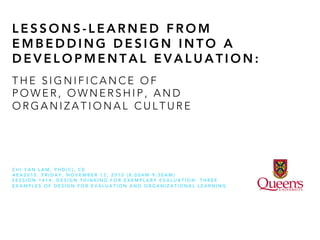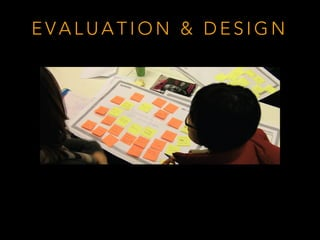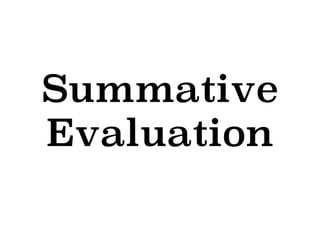Seu SlideShare está sendo baixado. ×

# Lessons-learned from embedding design into a developmental evaluation: The significance of power, ownership and organizational culture.

Anúncio
Anúncio
Anúncio
Anúncio
Anúncio
Anúncio
Anúncio
Anúncio
Anúncio
Anúncio
AnúncioCarregando em…3
×

1 de 16 Anúncio

# Lessons-learned from embedding design into a developmental evaluation: The significance of power, ownership and organizational culture.

Presented at the 2015 Annual Conference of the American Evaluation Association #eval15. ABSTRACT: Recent attempts at developmental evaluation (DE) are incorporating human-centered design (HCD) principles (Dorst, 2011; IDEO, n.d.) to facilitate program development. HCD promotes a design-oriented stance toward program development and articulates a set of values that focuses the evaluation beyond those ideals expressed by stakeholders. Embedding design into DE promises to offer a more powerful means to promoting program development beyond either approach alone. Yet, embedding design into DE introduces additional challenges. Drawing on a case study into a design-informed DE, this panelist discusses the tensions and challenges that arose as one developmental evaluator attempted to introduce design into a DE. Insights from the case study point to the importance of:

- Attending to power dynamics that could stifle or promote design integration; and,

- Evaluator sensitivity over the deep attachment program developers had over program decisions

These findings allude to the significance of organizational culture in enabling a design-informed DE.

Presented at the 2015 Annual Conference of the American Evaluation Association #eval15. ABSTRACT: Recent attempts at developmental evaluation (DE) are incorporating human-centered design (HCD) principles (Dorst, 2011; IDEO, n.d.) to facilitate program development. HCD promotes a design-oriented stance toward program development and articulates a set of values that focuses the evaluation beyond those ideals expressed by stakeholders. Embedding design into DE promises to offer a more powerful means to promoting program development beyond either approach alone. Yet, embedding design into DE introduces additional challenges. Drawing on a case study into a design-informed DE, this panelist discusses the tensions and challenges that arose as one developmental evaluator attempted to introduce design into a DE. Insights from the case study point to the importance of:

- Attending to power dynamics that could stifle or promote design integration; and,

- Evaluator sensitivity over the deep attachment program developers had over program decisions

These findings allude to the significance of organizational culture in enabling a design-informed DE.

Anúncio
Anúncio

### Lessons-learned from embedding design into a developmental evaluation: The significance of power, ownership and organizational culture.

1. 1. L E S S O N S - L E A R N E D F R O M E M B E D D I N G D E S I G N I N T O A D E V E L O P M E N T A L E V A L U A T I O N : C H I Y A N L A M , P H D ( C ) , C E A E A 2 0 1 5 . F R I D A Y , N O V E M B E R 1 3 , 2 0 1 5 ( 8 : 0 0 A M - 9 : 3 0 A M ) S E S S I O N 1 4 1 4 : D E S I G N T H I N K I N G F O R E X E M P L A R Y E V A L U A T I O N : T H R E E E X A M P L E S O F D E S I G N F O R E V A L U A T I O N A N D O R G A N I Z A T I O N A L L E A R N I N G T H E S I G N I F I C A N C E O F P O W E R , O W N E R S H I P , A N D O R G A N I Z A T I O N A L C U L T U R E
2. 2. E V A L U A T I O N & D E S I G N
3. 3. Summative Evaluation
4. 4. Formative Evaluation
5. 5. Developmental Evaluation • Program Development: ongoing shaping and re-shaping of a program in response to emerging evidence • Developmental Evaluation (Patton, 1994)
6. 6. (Patton, 2011) •  DE is laden with conceptual and practical challenges. (Not a criticism of the approach, but an indication of its complexity). ! •  What questions do you actually ask in a developmental evaluation?! Developmental Evaluation
7. 7. T H E D E V E L O P M E N T A L E V A L U A T I O N C A S E •  Faculty of Education •  Graduate-level Diploma and Professional Masters in Education •  To support the conceptualization, planning, and ‘decision-making’
8. 8. T A S K F O R C E a program “in theory” a program “in action”
9. 9. H O W M I G H T W E … D E V E L O P A P R O G R A M T H A T S U P P O R T S N O V I C E E D U C A T O R S I N E M B R A C I N G P R O F E S S I O N A L I N Q U I R Y T H R O U G H T E C H N O L O G Y ? a program in theory a program in action
10. 10. I N C O N V E N T I O N A L P L A N N I N G T E R M S … I N A D E S I G N F R A M E … H O W M I G H T W E … C O U R S E W O R K W H A T D O W E T E A C H ? W H A T A R E T H E L E A R N I N G O U T O C M E S ? C O N S T R U C T M E A N I N G F U L L E A R N I N G E X P E R I E N C E S T H A T E N G A G E O U R L E A R N E R S I N K N O W L E D G E C O N S T R U C T I O N ? L E A R N I N G M A N A G E M E N T S Y S T E M W H I C H S Y S T E M D O W E A D O P T ? L E V E R A G E T H E F U N C T I O N A L I T Y O F L M S A N D E X T E R N A L T O O L S T O E N A B L E P A R T I C U L A R K I N D S O F L E A R N I N G ? M A R K E T I N G W H A T C H A N N E L S A N D T O O L S D O W E U S E T O S E L L T H E P R O G R A M ? B E S T H E L P P O T E N T I A L S T U D E N T S L E A R N A B O U T T H E F E A T U R E S A N D V A L U E O F O U R P R O G R A M ? M A R K E T S W H O D O W E T A R G E T ? W H O W O U L D ‘ B U Y ’ T H I S ? I D E N T I F Y P R O G R A M U S E R S W H O C O U L D B E N E F I T F R O M T H I S ?
11. 11. U S E R - C E N T E R E D P E R S P E C T I V E T H I N K C R E A T I V E L Y P R O D U C T I V E P R O B L E M A T I Z I N G
12. 12. U S E R - C E N T E R E D P E R S P E C T I V E T H I N K C R E A T I V E L Y P R O D U C T I V E P R O B L E M A T I Z I N G R E A L I T Y - T E S T H O L D O U R I D E A S L I G H T L Y
13. 13. P O W E R •  Design-informed DE requires buy-in from leaders. •  necessitates participation from all levels •  the role of an evaluator, is sometimes, to rebalance and shift the power
14. 14. O W N E R - S H I P •  investment in the design/development process means that staff can feels strongly about the ideas •  when the idea doesn’t work in reality, it can be difficult to accept •  speaking truth requires a delicate touch
15. 15. O R G A N I - Z A T I O N A L C U L T U R E •  Attention to process is not enough. •  Structure matters •  Traditional structure: •  budgeting •  committee •  cross-functional team •  Consider “intrapreneurship" •  flexibility to pivot and change ideas •  resource to test and fail. •  permission to fail
16. 16. Q U E S T I O N S ?